## Z Transform Sinusoidal Response

Ele 541 Electronic Testing

Sinusoidal Response

Consider the response of a filter to the complex simusoidal signal x(k).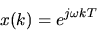The convolution summation describes the response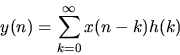Plugging in the sinusoidal input x(k)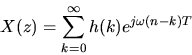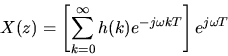where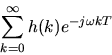is H(z), the z-transform of h(k) with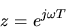. Therefore lettingin the unit pulse response of a filter, H(z), allows us to evaluate the sinusoidal response of the filter. More information on
[Sequences and filters] [Common Sequences ] [Delay] [Sinusoidal Response]